American Journal of Physics and Applications
Volume 4, Issue 2, March 2016, Pages: 64-70

Bounding the Greybody Factor, Temperature and Entropy of Black Holes in dRGT Massive Gravity

Tritos Ngampitipan1, *, Petarpa Boonserm2, Pitayuth Wongjun3

1Faculty of Science, Chandrakasem Rajabhat University, Bangkok, Thailand

2Department of Mathematics and Computer Science, Faculty of Science, Chulalongkorn University, Bangkok, Thailand

3The institute for Fundamental Study, Naresuan University, Phitsanulok, Thailand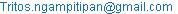(T. Ngampitipan)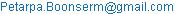(P. Boonserm)(P. Wongjun)

*Corresponding author

Tritos Ngampitipan, Petarpa Boonserm, Pitayuth Wongjun. Bounding the Greybody Factor, Temperature and Entropy of Black Holes in dRGT Massive Gravity. American Journal of Physics and Applications. Vol. 4, No. 2, 2016, pp. 64-70. doi: 10.11648/j.ajpa.20160402.15

Received: February 28, 2016; Accepted: March 24, 2016; Published: April 9, 2016

Abstract: A black hole greybody factor is the quantum quantity of a black hole. It is the fraction of Hawking radiation that can reach spatial infinity. The greybody factor may contain the necessary information to support the theory of quantum gravity. An understanding of the greybody factor helps us gain insight, not only into the nature of the black hole itself, but also into the theory of quantum gravity, which is currently being developed via numerous attempts. In this paper, we calculate the bound on the greybody factor for scalar field emitted from black holes in dRGT massive gravity. The bound on the reflection probability is also determined. Moreover, the effects of massive gravity on the greybody factors are explored. The results show that the bound on the greybody factor for the dRGT black holes is less than the bound for the Schwarzschild-de-Sitter black hole. The Hawking temperature is also calculated, both in the dRGT case and in the Schwarzschild-de-Sitter case. It is found that the Hawking temperature of the dRGT black hole is higher than that of the Schwarzschild-de-Sitter black hole. The increase in the Hawking temperature probably results from the mass of graviton. Finally, the black hole entropy is also determined. We found that the entropy of the Schwarzschild-de-Sitter black hole is more than the entropy of the dRGT black hole.

Keywords: dRGT Black Hole, Greybody Factor, Hawking Temperature, Massive Gravity, Modified General Relativity

Contents

1. Introduction

Black hole greybody factors have been of great interest in the field of quantum physics. Studying greybody factors can give an understanding of the quantum structure of black holes, which can potentially lead to an understanding of quantum gravity, and the quantum behavior of gravitational field. Quantum gravity is one of the unsolved problems of theoretical physics. This is because quantum theory is not in consistent with general relativity. A classic example of systems that have to be described by both quantum theory and general relativity is black hole.

The existence of black hole has been predicted by general relativity. It is a region of spacetime that has such a strong gravitational field that nothing can escape, not even light. If the quantum effects are taken into account, black holes can emit thermal radiation. This radiation became known as Hawking radiation . While Hawking radiation propagates to an observer away from a black hole, it is modified by the curvature of spacetime resulting from the black hole itself. Therefore, only a fraction of Hawking radiation can arrive at an observer. This fraction is called the greybody factor.

Derivation of the greybody factor has been conducted in various ways. Some use the matching techniques to obtain the approximate greybody factor [2-4], while some use the WKB approximation [5-7]. Moreover, a new method to derive the greybody factor without approximation has also been developed. This new method requires the calculation of the bound on the greybody factor [8-10]. The bound is relatively simple to derive and can provide a qualitative understanding of black holes.

Recently, massive gravity theories have been developed . Massive gravity is the modification of general relativity by re-interpreting the terms of interaction as graviton mass. This theory can account for the accelerated expansion of the universe, invalidating the concept of cosmological constant. The de Rham, Gabadadze and Tolley (dRGT) massive gravity is constructed in a way that the ghost field disappears [12-13]. It is possible for black holes to exist in the dRGT massive gravity [14-15].

P. Boonserm and M. Visser  applied the rigorous bounds on the greybody factor to Schwarzschild black holes. These bounds can be obtained from the Regge-Wheeler equation which governs the motion of any type of particles in a non-rotational spacetime. The results show that the bounds are valid for all frequencies. At high frequencies, the potential from the Schwarzschild black hole is transparent regardless of the type of Hawking radiation.

T. Ngampitipan and P. Boonserm  applied the rigorous bounds on the greybody factor to non-rotating black holes for scalar emission. The results show that the charges of the Reissner-Nordstrom black holes and the charged dilatonic black holes behave as barriers. A higher number of spacetime dimensions can prevent Hawking radiation from reaching spatial infinity. Moreover, the cosmological constant can increase the bounds on the greybody factor.

P. Boonserm, T. Ngampitipan, and M. Visser  derived a general form of the Regge-Wheeler equation for scalar, vector, and axial spin 2 perturbations on an arbitrary static spherically symmetric spacetime. The black holes in this spacetime are called dirty black holes. We applied the rigorous bounds on the greybody factor to these dirty black holes. We also applied the rigorous bounds on the greybody factor for scalar emission to the Kerr-Newman black holes, ones with charges and rotation . These bounds can be obtained from the Teukolsky equation, which governs the motion of any type of particle in a rotational spacetime. We obtained the bounds on the greybody factor for the zero-angular-momentum modes, the non-super-radiant modes, and the super-radiant modes. The results show that the bounds increase if the frequencies increase. However, the bounds also increase if the angular momentum and the electric charge decrease.

Furthermore, Boonserm et. al  obtained the rigorous bounds on the greybody factor for the Myers-Perry black holes in the case of scalar field excitation. Myers-Perry black holes are uncharged rotating black holes in higher dimensions. In four dimensions, the Myers-Perry black holes reduce to Kerr black holes. The bounds from Myers-Perry black holes can also be derived from the Teukolsky equation. We calculated these bounds for the zero-angular-momentum modes, the non-super-radiant modes, and the super-radiant modes. Moreover, we obtained the emission rates for the super-radiant modes. The results show that the bounds on the greybody factors decrease in higher dimensions. This type of black holes may be created in future experiments such as at the LHC [21-31]. Then, the bounds derived from the Myers-Perry black holes can be useful in describing the results of those experiments.

In this paper, we study the dRGT spacetime in section 2. In section 3, we derive the equation of motion of the scalar field emitted from the dRGT black holes. In the next section, we calculate the bound on the greybody factor for scalar field emitted from black holes in dRGT massive gravity. The effects of massive gravity on the greybody factors will be explored. The comparisons between the angular momentum and the potential, as well as the comparisons between the reflection and transmission probabilities are also made. In section 5, we calculate the Hawking temperature, both in the dRGT black hole and in the Schwarzschild-de-Sitter black hole. Finally, the conclusions are drawn in section 6.

2. dRGT Spacetime

The metric of the static and spherically symmetric spacetime in massive gravity is given by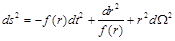(1)

where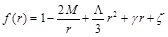(2)

We can express the parameters appearing in the above equation as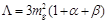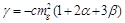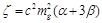(3)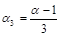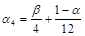where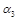and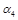are the free parameters of the theory and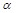and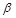are the parameters related toand. Moreover,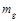is the graviton mass. For the special case,,, the dRGT solution reduces to the Schwarzschild-de-Sitter solution [11, 32]. In this case, the above parameters are given by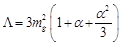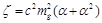(4)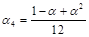3. Massless Scalar Field Emitted from the dRGT Black Hole

When the dRGT black hole emits a scalar wave, its equation of motion takes the form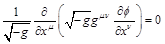(5)

To solve the above equation, we use the separation of variables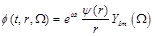(6)

The radial equation of motion can be obtained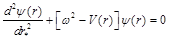(7)

The tortoise coordinate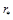is defined by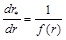(8)

and the dRGT potential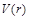is given by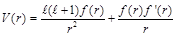(9)

The potential is plotted as shown in Figure 1 and Figure 2.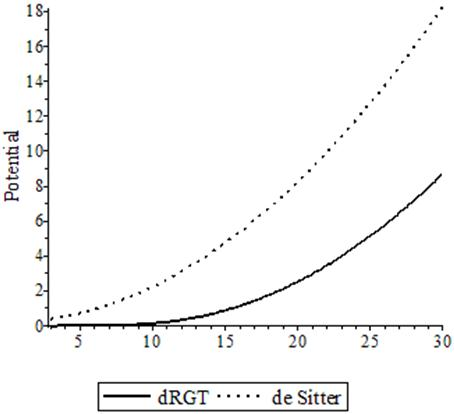Figure 1. The dRGT potential versus the de Sitter potential.Figure 2. The dRGT potential for various angular momentum (l = 0, l = 1, l = 2, l =3, and l = 4).

We can see that in the beginning the potential for the higher angular momentum is greater than the potential for the lower angular momentum. In the intermediate region, the relation reverses. That is, when the angular momentum increases, the potential decreases. After that, the relation is the same as the beginning. The potential for the highest angular momentum is the highest.

4. The Rigorous Bound on the Transmission Probability

In order to calculate the transmission probability, we have to solve the Teukolsky equation for the given potential. In the dRGT case, it seems that no exact solution exists. To handle this problem, we introduce the new method of the rigorous bound to find the transmission probability. In [8-10], the rigorous bound on the transmission probability is given by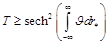(10)

where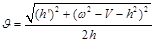(11)

and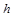is a positive function. For simplicity, we choose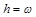. Therefore,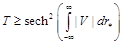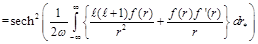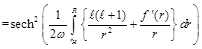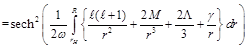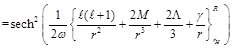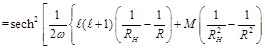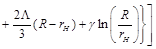(12)

The effect of massive gravity on the rigorous bound on transmission probability is plotted as shown in Figure 3.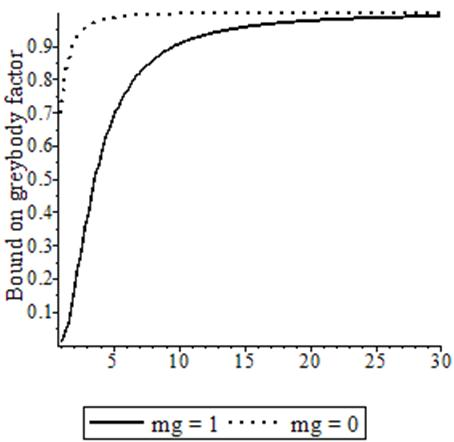Figure 3. The effect of massive gravity on the rigorous bound on transmission probability.

From the law of conservation, the sum of the reflection probability and the transmission probability is in unity. Therefore, with the rigorous bound on transmission probability, we can derive the reflection probability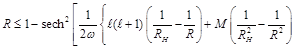(13)

The relationship between the reflection probability and the transmission probability for the dRGT case and the Schwarzschild-de-Sitter case are plotted as shown in Figure 4 and Figure 5, respectively.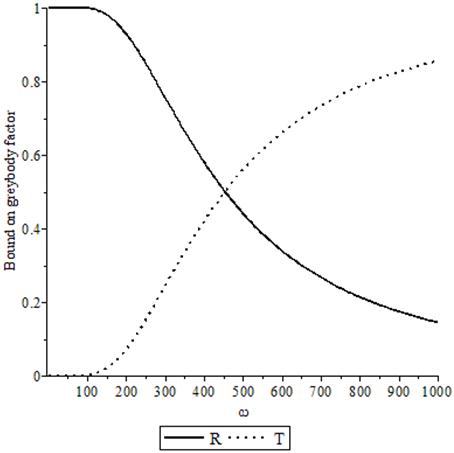Figure 4. The relationship between the reflection probability and the transmission probability for the dRGT case.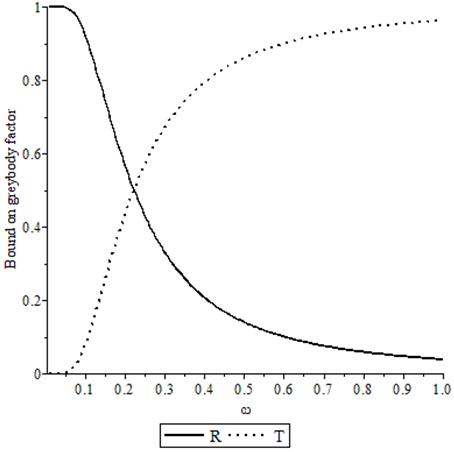Figure 5. The relationship between the reflection probability and the transmission probability for the Schwarzschild-de-Sitter case.

From these figures, we can see that the decrease in the rigorous bound on the greybody factor (corresponding to the increase in the rigorous bound on the reflection probability) occurs when the wave’s energy increases.

In summary, the relationship between the reflection and the transmission probabilities, and the potential can be shown in Table 1.

Table 1. The potential and the reflection and transmission probabilities for various angular momentum.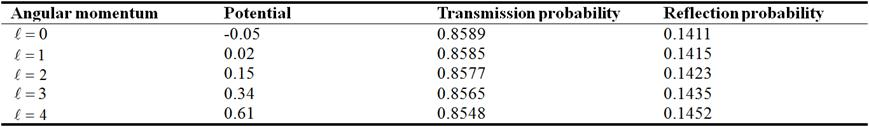We can see that when the angular momentum increases, the potential and the reflection probability also increase, while the transmission probability decreases. From the table, we notice that the potential for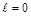can be negative. The negative value of the potential indicates that the curvature of spacetime is different from the positive value of the potential .

5. Hawking Temperature and Entropy

5.1. Hawking Temperature

In this section, we study the thermodynamics of black holes in dRGT massive gravity. The black hole thermodynamics is associated with the temperature, the entropy, the Helmholtz free energy, and the heat capacity. In this paper, we pay attention to the temperature of Hawking radiation (called Hawking temperature). The Hawking temperature can be expressed in terms of surface gravity (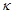)(14)

where the surface gravity is given by(15)

Therefore, for black holes in dRGT massive gravity, the Hawking temperature is given by(16)

For the Schwarzschild-de-Sitter black holes, the Hawking temperature is given by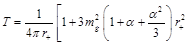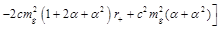(17)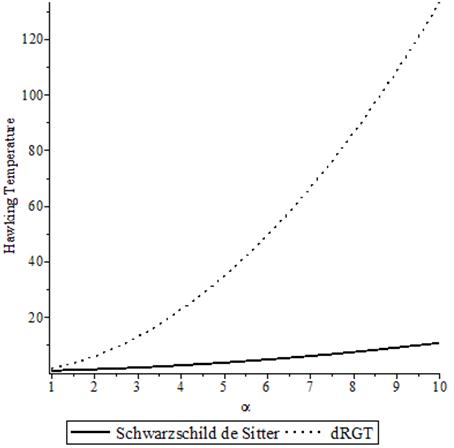Figure 6. The Hawking temperature between the dRGT case and the Schwarzschild-de-Sitter.

Comparison of the Hawking temperature between the dRGT case and the Schwarzschild-de-Sitter is made as shown in Figure 6.

From Figure 5, we can see that for the specific parameters, the Hawking temperature of dRGT black hole is higher than that of the Schwarzschild-de-Sitter black hole. This may be because when the graviton becomes massive, the temperature is raised. However, for other choices of parameters, the results may be different.

5.2. Entropy

One of the important quantities in thermodynamics is entropy. For black hole systems, entropy is also significant for black hole thermodynamics. In this section, we will calculate the entropy of the dRGT black hole and the Schwarzschild-de-Sitter black hole. The entropy is related to the Hawking temperature by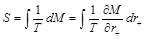(18)

where T is the Hawking temperature, M is the black hole mass, and r+ is the horizon radius. The above integral can be calculated and we obtain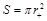(19)

The horizon radius of dRGT black hole and Schwarzschild-de-Sitter black hole can be found from the solution of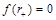(20)

Solving this equation, we obtain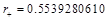for the horizon radius of the dRGT black hole and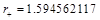for the horizon radius of the Schwarzschild-de-Sitter black hole. Therefore, the entropy is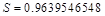and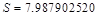for the dRGT black hole and the Schwarzschild-de-Sitter black hole, respectively. That is, the entropy of the Schwarzschild-de-Sitter black hole is approximately 8 times greater than the entropy of the dRGT black hole. Notice that the black hole entropy depends on the horizon radius.

6. Conclusion

In this paper, we calculated the bound on the greybody factor for scalar field emitted from black holes in dRGT massive gravity. The bound on the reflection probability was also calculated. The effects of massive gravity on the greybody factors were also explored. The results show that the rigorous bound on the greybody factor for the dRGT black hole is less than that of the Schwarzschild-de-Sitter black hole. When the angular momentum increases, the potential and the rigorous bound on the reflection probability also increase. However, the rigorous bound on the transmission probability decreases. Moreover, we calculated the Hawking temperature, both for the dRGT black hole and the Schwarzschild-de-Sitter black hole. It was found that the Hawking temperature of the dRGT black hole is higher than that of the Schwarzschild-de-Sitter black hole. The increase in the Hawking temperature probably comes from graviton mass. In addition, the black hole entropy was also investigated. The results indicate that the entropy of the Schwarzschild-de-Sitter black hole is more than the entropy of the dRGT black hole.

Acknowledgements

This project was funded by the Ratchadapisek Sompoch Endowment Fund, Chulalongkorn University (Sci-Super 2014-032), by a grant for the professional development of new academic staff from the Ratchadapisek Somphot Fund at Chulalongkorn University, by the Thailand Research Fund (TRF), and by the Office of the Higher Education Commission (OHEC), Faculty of Science, Chulalongkorn University. PB was additionally supported by a scholarship from the Royal Government of Thailand. TN was also additionally supported by a scholarship from the Development and Promotion of Science and Technology talent project (DPST). PW was also additional supported by the Naresuan University Research Fund through grant No. R2557C083 and the Thailand Toray Science Foundation (TTSF) science and technology research grant.

References

1. S. W. Hawking, "Particle creation by black holes", Commun. Math. Phys. 43, 199 (1975).
2. S. Fernando, "Greybody factors of charged dilaton black holes in 2 + 1 dimensions", Gen. Relativ. Gravit. 37, 461-481 (2005).
3. W. Kim and J. J. Oh, "Greybody Factor and Hawking Radiation of Charged Dilatonic Black Holes", JKPS 52, 986 - 991 (2008).
4. J. Escobedo, "Greybody Factors Hawking Radiation in Disguise", Master’s Thesis, University of Amsterdam (2008). 6
5. M. K. Parikh and F. Wilczek, "Hawking Radiation as Tunneling", Phys. Rev. Lett. 85, 5042-5045 (2000) [arXiv: hep-th/9907001].
6. C. H. Fleming, "Hawking Radiation as Tunneling", (2005) http://www.physics.umd.edu/grt/taj/776b/fleming. pdf.
7. P. Lange, "Calculation of Hawking Radiation as Quantum Mechanical Tunneling", Thesis, Uppsala Universitet (2007).
8. M. Visser, "Some general bounds for 1-D scattering", Phys. Rev. A 59, 427438 (1999) [arXiv: quant-ph/9901030].
9. P. Boonserm and M. Visser, "Bounding the Bogoliubov coefficients", Annals Phys. 323, 2779 - 2798 (2008) [arXiv: 0801.0610 [quant-ph]].
10. P. Boonserm, "Rigorous Bounds on Transmission, Reflection, and Bogoliubov Coefficients", Ph. D. Thesis, Victoria University of Wellington (2009) [arXiv: 0907.0045 [mathph]].
11. S. G. Ghosh, L. Tannukij, and P. Wongjun, "A class of black holes in dRGT massive gravity and their thermodynamical properties", [arXiv: 1506.07119 [gr-qc]].
12. C. de Rham, G. Gabadadze, "Generalization of the Fierz-Pauli action", Phys. Rev. D 82, 044020 (2010) [arXiv: 1007.0443 [hep-th]].
13. C. de Rham, G. Gabadadze, and A. J. Tolley, "Resummation of Massive Gravity", Phys. Rev. Lett. 106, 231101 (2011) [arXiv: 1011.1232 [hep-th]].
14. M. S. Volkov, "Self-accelerating cosmologies and hairy black holes in ghost-free bigravity and massive gravity", Class. Quant. Grav. 30, 184009 (2013) [arXiv: 1304.0238 [hep-th]].
15. G. Tasinato, K. Koyama, and G. Niz, "Exact solutions in massive gravity", Class. Quant. Grav. 30, 184002 (2013) [arXiv: 1304.0601 [hep-th]].
16. P. Boonserm and M. Visser, "Bounding the greybody factors for Schwarzchild black holes", Phys. Rev. D 78, 101502 (2008) [arXiv: 0806.2209 [gr-qc]].
17. T. Ngampitipan and P. Boonserm, "Bounding the greybody factors for non-rotating black holes", Int. J. Mod. Phys. D 22, 1350058 (2013) [arXiv: 1211.4070 [math-ph]].
18. P. Boonserm, T. Ngampitipan and M. Visser, "Regge-Wheeler equation, linear stability and greybody factors for dirty black holes", Phys. Rev. D 88, 041502 (2013) [arXiv: 1305.1416 [gr-qc]].
19. P. Boonserm, T. Ngampitipan, and M. Visser, "Bounding the greybody factors for scalar field excitations of the Kerr-Newman spacetime", J. High Energy Phys. 113 (2014) [arXiv: 1401.0568 [gr-qc]].
20. P. Boonserm, A. Chatrabhuti, T. Ngampitipan, and M. Visser, "Greybody factors for Myers–Perry black holes", J. Math. Phys. 55, 112502 (2014) [arXiv: 1405.5678[gr-qc]].
21. P. Kanti, "Black holes in theories with large extra dimensions: a review" Int. J. Mod. Phys. A 19, 4899 (2004) [arXiv: hep-ph/0402168].
22. M. Cavaglia, "Black hole and brane production in TeV gravity: A review", Int. J. Mod. Phys. A 18, 1843 (2003) [arXiv: hep-ph/0210296].
23. G. Landsberg, "Black holes at future colliders and in cosmic rays", Eur. Phys. J. C 33, 927 (2004) [arXiv: hep-ex/0310034].
24. K. Cheung, "Collider phenomenology for a few models of extra dimensions", [arXiv: hep-ph/0409028].
25. S. Hossenfelder, "What black holes can teach us", Focus on Black Hole Research 155, (2005) [arXiv: hep-ph/0412265].
26. C. M. Harris, "Physics beyond the standard model: Exotic leptons and black holes at future colliders", Ph. D. Thesis, University of Cambridge (2003) [arXiv: hep-ph/0502005].
27. A. S. Majumdar and N. Mukherjee, "Braneworld black holes in cosmology and astrophysics", Int. J. Mod. Phys. D 14, 1095 (2005) [arXiv: astro-ph/0503473].
28. J. Man and H. Cheng, "The greybody factor for scalar fields in the Schwarzschild spacetime with an f(R) global monopole", [arXiv: 1402.4215 [hep-th]].
29. P. Kanti and J. M. Russell, "Calculable corrections to brane black hole decay. II. Greybody factors for spin 1/2 and 1", Phys. Rev. D 67, 104019 (2003) [arXiv: hep-ph/0212199].
30. O. Efthimiou, "Graviton emission from a Schwarzschild black hole in the presence of extra dimensions", J. Phys: Conf. Ser. 68, 012024 (2007) [arXiv: hep-th/0609144].
31. J. V. Rocha, "Evaporation of large black holes in AdS: greybody factor and decay rate", J. High Energy Phys. 08, 027 (2009) [arXiv: 0905.4373 [hep-th]].
32. S. G. Ghosh and S. D. Maharaj, "A class of black holes in dRGT massive gravity and their thermodynamical properties" Phys. Rev. D 89, no. 8, 084027 (2014) [arXiv: 1506.07119 [gr-qc]].
33. S. Caroll, Spacetime and Geometry: An Introduction to General Relativity, Addison-Wesley 2004.

 Contents 1. 2. 3. 4. 5. 5.1. 5.2. 6.
Article ToolsAbstractPDF(775K)